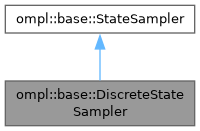ompl::base::DiscreteStateSampler Class Reference

State space sampler for discrete states. More...

#include <ompl/base/spaces/DiscreteStateSpace.h>

Inheritance diagram for ompl::base::DiscreteStateSampler:[legend]

## Public Member Functions

DiscreteStateSampler (const StateSpace *space)
Constructor.

void sampleUniform (State *state) override
Sample a state.

void sampleUniformNear (State *state, const State *near, double distance) override
Sample a state near another, within a neighborhood controlled by a distance parameter. More...

void sampleGaussian (State *state, const State *mean, double stdDev) override
Sample a state using a Gaussian distribution with given mean and standard deviation (stdDev). More...Public Member Functions inherited from ompl::base::StateSampler
StateSampler (const StateSampler &)=delete

StateSampleroperator= (const StateSampler &)=delete

StateSampler (const StateSpace *space)
Constructor.Protected Attributes inherited from ompl::base::StateSampler
const StateSpacespace_
The state space this sampler samples.

RNG rng_
An instance of a random number generator.

## Detailed Description

State space sampler for discrete states.

Definition at line 111 of file DiscreteStateSpace.h.

## ◆ sampleGaussian()

 void ompl::base::DiscreteStateSampler::sampleGaussian ( State * state, const State * mean, double stdDev )
overridevirtual

Sample a state using a Gaussian distribution with given mean and standard deviation (stdDev).

As with sampleUniform, the implementation of sampleGaussian is specific to the derived class and few assumptions can be made about the distance between state and mean.

Implements ompl::base::StateSampler.

Definition at line 56 of file DiscreteStateSpace.cpp.

## ◆ sampleUniformNear()

 void ompl::base::DiscreteStateSampler::sampleUniformNear ( State * state, const State * near, double distance )
overridevirtual

Sample a state near another, within a neighborhood controlled by a distance parameter.

Typically, StateSampler-derived classes will return in state a state that is uniformly distributed within a ball with radius distance defined by the distance function from the corresponding state space. However, this is not guaranteed. For example, the default state sampler for the RealVectorStateSpace returns samples uniformly distributed using L_inf distance, while the default distance function is L_2 distance.

Implements ompl::base::StateSampler.

Definition at line 48 of file DiscreteStateSpace.cpp.

The documentation for this class was generated from the following files: